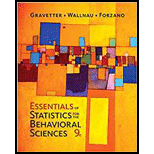Chapter 5, Problem 17P### Essentials of Statistics for The B...

9th Edition
Frederick J Gravetter + 2 others
ISBN: 9781337098120

#### Solutions

Chapter
Section### Essentials of Statistics for The B...

9th Edition
Frederick J Gravetter + 2 others
ISBN: 9781337098120
Textbook Problem

# For a sample with a mean of M - 63, a score of X = 54 corresponds to z = –0.75. What is the sample standard deviation?

To determine

To Find: The sample standard deviation.

Explanation

Given Info:

The sample has mean M=63 , score of X=54 and z score = –0.75.

Calculation:

Use the formula for z score to find the sample standard deviation is,

z=XMss=XMz

Substitute the value 54 for X, 63 for M and -0

### Still sussing out bartleby?

Check out a sample textbook solution.

See a sample solution

#### The Solution to Your Study Problems

Bartleby provides explanations to thousands of textbook problems written by our experts, many with advanced degrees!

Get Started

## Additional Math Solutions

#### Find more solutions based on key concepts